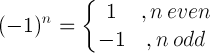# Exponent rules

Exponent rules, laws of exponent and examples.

## What is an exponent

The base a raised to the power of n is equal to the multiplication of a, n times:

a n = a × a × ... × a

n times

a is the base and n is the exponent.

#### Examples

31 = 3

32 = 3 × 3 = 9

33 = 3 × 3 × 3 = 27

34 = 3 × 3 × 3 × 3 = 81

35 = 3 × 3 × 3 × 3 × 3 = 243

## Exponents rules and properties

Rule name Rule Example
Product rules a na m = a n+m 23 ⋅ 24 = 23+4 = 128
a nb n = (a b) n 32 ⋅ 42 = (3⋅4)2 = 144
Quotient rules a n / a m = a n-m 25 / 23 = 25-3 = 4
a n / b n = (a / b) n 43 / 23 = (4/2)3 = 8
Power rules (bn)m = bn⋅m (23)2 = 23⋅2 = 64
bnm = b(nm) 232 = 2(32)= 512
m√(bn) = b n/m 2√(26) = 26/2 = 8
b1/n = nb 81/3 = 38 = 2
Negative exponents b-n = 1 / bn 2-3 = 1/23 = 0.125
Zero rules b0 = 1 50 = 1
0n = 0 , for n>0 05 = 0
One rules b1 = b 51 = 5
1n = 1 15 = 1
Minus one rule(-1)5 = -1
Derivative rule (xn)' = nx n-1 (x3)' = 3⋅x3-1
Integral rule xndx = xn+1/(n+1)+C x2dx = x2+1/(2+1)+C

## Exponents product rules

anam = an+m

#### Example:

23 ⋅ 24 = 23+4 = 27 = 2⋅2⋅2⋅2⋅2⋅2⋅2 = 128

anbn = (a b)n

#### Example:

32 ⋅ 42 = (3⋅4)2 = 122 = 12⋅12 = 144

## Exponents quotient rules

an / am = an-m

#### Example:

25 / 23 = 25-3 = 22 = 2⋅2 = 4

#### Quotient rule with same exponent

an / bn = (a / b)n

#### Example:

43 / 23 = (4/2)3 = 23 = 2⋅2⋅2 = 8

## Exponents power rules

(an) m = a n⋅m

#### Example:

(23)2 = 23⋅2 = 26 = 2⋅2⋅2⋅2⋅2⋅2 = 64

a nm = a (nm)

#### Example:

232 = 2(32) = 2(3⋅3) = 29 = 2⋅2⋅2⋅2⋅2⋅2⋅2⋅2⋅2 = 512

m√(a n) = a n/m

#### Example:

2√(26) = 26/2 = 23 = 2⋅2⋅2 = 8

## Negative exponents rule

b-n = 1 / bn

#### Example:

2-3 = 1/23 = 1/(2⋅2⋅2) = 1/8 = 0.125

Exponent calculator »

Currently, we have around 1975 calculators, conversion tables and usefull online tools and software features for students, teaching and teachers, designers and simply for everyone.